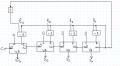# Arithmetic - Absolut Value

#### GastonJam

Joined Jun 25, 2020
11
I want to construct a circuit that calculates the absolute value of a signed 4-bit number in two's complement!

So the logic here : assuming we have B = b3 b2 b1 b0 . If b3 is '1' then then b is already in 2s complemnt and should be assigned directly to the output else if b3 is equal to '0' then we should convert B to 2s complement .

This is what I did :Then a question came where I should show the correctness of your circuit using the definitions of the two's complement representation [.] and the binary representation (.)! With a possibility to use the lemma rule
$$2^n = 1 + \sum_{i=0}^{n-1} 2^i$$
and the definition ¬x = (1 − x).

How am I supposed to verify this circuit using these 2 rules ?

Last edited: